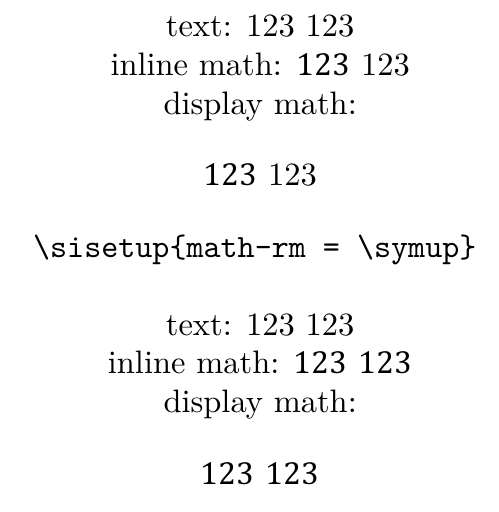# #StackBounty: #fonts #fontspec #siunitx #unicode-math fonts / siunitx: Understanding how "detect-all" and "detect-inline…

### Bounty: 50

For the following, I need to

1- understand why neither `detect-all` nor `detect-inline-family=math` doesn’t make `num` print the same font as the surrounding inline math, and the only way to do so is through `math-rm = symup` as suggested by this answer.

2- understand why I need to load the option `math-rm = symup` in order to make `num` copy the document default math font.

3- know if it is possible to make the default math font-weight as light as the surrounding normal text since it looks a bit bolder for my eyes.``````documentclass[14pt]{article}

usepackage[no-math]{fontspec}
setmainfont{Latin Modern Roman}

usepackage{unicode-math}
setmathfont[math-style=ISO]{Cambria Math}

usepackage[
detect-all,
detect-inline-family=math
]{siunitx}

begin{document}
centering
text: 123 num{123}\
inline math: \$123~num{123}\$\
display math: [123~num{123}]

verb|sisetup{math-rm = symup}|
sisetup{math-rm = symup} % https://tex.stackexchange.com/a/438342/2288

vspace{baselineskip}
text: 123 num{123}\
inline math: \$123~num{123}\$\
display math: [123~num{123}]
end{document}
``````

Get this bounty!!!

This site uses Akismet to reduce spam. Learn how your comment data is processed.